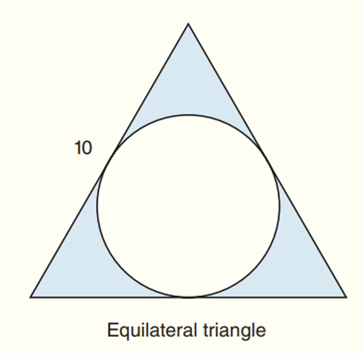Chapter 8.CR, Problem 31CR### Elementary Geometry for College St...

6th Edition
Daniel C. Alexander + 1 other
ISBN: 9781285195698

#### Solutions

Chapter
Section### Elementary Geometry for College St...

6th Edition
Daniel C. Alexander + 1 other
ISBN: 9781285195698
Textbook Problem
1 views

# Find the exact areas of the shaded regions in Exercises 27 to 31.To determine

To find:

The exact areas of the shaded regions.

Explanation

Calculation:

Consider the following diagram as shown below:

ABC is the equilateral triangle.

In equilateral triangle, the circle touches at the midpoint of the sides of the triangle.

In equilateral triangle, the median passes through the origin of the circle and origin becomes centroid of the triangle.

Centroid divides the median in ration 2:1 from the vertex.

In equilateral; triangle, the median becomes perpendicular bisector of the triangle.

That is BD¯=DC¯

BC¯=BD¯+DC¯10=2(BD¯) [Since BD¯=DC¯]BD¯=102BD¯=5

Now find the length of the median AD¯

Use Pythagorean Theorem to the right angled triangle ADB

### Still sussing out bartleby?

Check out a sample textbook solution.

See a sample solution

#### The Solution to Your Study Problems

Bartleby provides explanations to thousands of textbook problems written by our experts, many with advanced degrees!

Get Started

#### Find limx1x2+4x+3x2+3x+2

Applied Calculus for the Managerial, Life, and Social Sciences: A Brief Approach

#### Evaluate the expression sin Exercises 116. (32)3

Finite Mathematics and Applied Calculus (MindTap Course List)

#### True or False: is a convergent series.

Study Guide for Stewart's Single Variable Calculus: Early Transcendentals, 8th